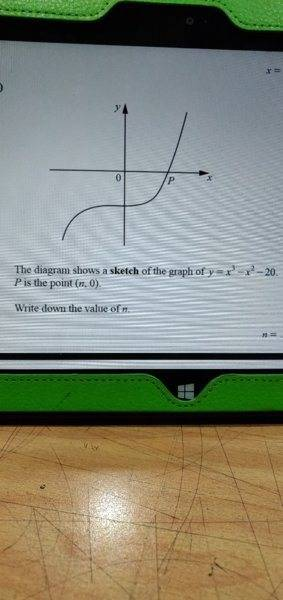# How do I find the value of n?

• lioric

## Homework StatementHow do I find the value of n?

## Homework Equations

I know that when it crosses x-axis y = 0
X3 - X2 - 20 =0
But don't know how to solve it from here

## The Attempt at a Solution

The marking scheme says 3.0 or 3.1
I couldn't get the answer even by substituting
I know I'm doing something stupid

#### Attachments

Have you googled "how to solve a cubic equation" ?

The answer was there in the previous part
Thank you

@lioric, what's the complete problem? In the image you posted, there is no "n".

@lioric, what's the complete problem? In the image you posted, there is no "n".
Look closer.Squint like Clint

#### Attachments

Last edited:
•berkeman
I was already squinting, looking at that unclear and incomplete image. By "there is no n" I meant that the equation doesn't involve n.

Look closer.

View attachment 229330
Squint like Clint
When I looked at it the first time (on my phone) there was no n. When I looked at it later (on my laptop) there was, magically, an n.

If the answer is desired as a decimal, it seems that it is an infinite decimal, hence cannot be written down exactly. Thus the simplest way to approximate this decimal to a few places is to just plug in values. when x=2, we get 8 - 4 -20 = -16. when x=3 we get 27 - 9 -20 = -2. so we re getting closer. when x = 4 we get 64 - 16 - 20 = 28, so we are getting further away. hence the answer lies between 3 and 4, so next try 3.1 and then 3.2, etc..., and stop when you get a positive result. then if say 3.1 gives a negative result and 3.2 gives a positive result, you can choose the one that gives a result closer to zero, as a first decimal place approximation.

•